Courses

# Chemical Potential (Part -1)- Thermodynamic and Statistical Physics, CSIR-NET Physical Sciences Physics Notes | EduRev

## Physics for IIT JAM, UGC - NET, CSIR NET

Created by: Akhilesh Thakur

## Physics : Chemical Potential (Part -1)- Thermodynamic and Statistical Physics, CSIR-NET Physical Sciences Physics Notes | EduRev

The document Chemical Potential (Part -1)- Thermodynamic and Statistical Physics, CSIR-NET Physical Sciences Physics Notes | EduRev is a part of the Physics Course Physics for IIT JAM, UGC - NET, CSIR NET.
All you need of Physics at this link: Physics

In thermodynamics, chemical potential of a species, is a form of energy that can be absorbed or released during a chemical reaction or phase transition due to a change of the particle number of the given species. The chemical potential of a species in a mixture is defined as the rate of change of a free energy of a thermodynamic system with respect to the change in the number of atoms or molecules of the species that are added to the system. Thus, it is the partial derivative of the free energy with respect to the amount of the species, all other species' concentrations in the mixture remaining constant. The molar chemical potential is also known as partial molar free energy. When both temperature and pressure are held constant, chemical potential is the partial molar Gibbs free energy. At chemical equilibrium or in phase equilibrium the total sum of the product of chemical potentials and stoichiometric coefficients is zero, as the free energy is at a minimum.

In semiconductor physics, the chemical potential of a system of electrons at a temperature of zero Kelvin is known as the Fermi energy.

Overview

Particles tend to move from higher chemical potential to lower chemical potential. In this way, chemical potential is a generalization of "potentials" in physics such as gravitational potential. When a ball rolls down a hill, it is moving from a higher gravitational potential (higher internal energy thus higher potential for work) to a lower gravitational potential (lower internal energy). In the same way, as molecules move, react, dissolve, melt, etc., they will always tend naturally to go from a higher chemical potential to a lower one, changing the particle number, which is conjugate variable to chemical potential.

A simple example is a system of dilute molecules diffusing in a homogeneous environment. In this system, the molecules tend to move from areas with high concentration to low concentration, until eventually the concentration is the same everywhere.

The microscopic explanation for this is based in kinetic theory and the random motion of molecules. However, it is simpler to describe the process in terms of chemical potentials: For a given temperature, a molecule has a higher chemical potential in a higher-concentration area, and a lower chemical potential in a low concentration area. Movement of molecules from higher chemical potential to lower chemical potential is accompanied by a release of free energy. Therefore, it is a spontaneous process.

Another example, not based on concentration but on phase, is a glass of liquid water with ice cubes in it. Above 0 °C, an H2O molecule that is in the liquid phase (liquid water) has a lower chemical potential than a water molecule that is in the solid phase (ice). When some of the ice melts, H2O molecules convert from solid to liquid where their chemical potential is lower, so the ice cubes shrink. Below 0 °C, the molecules in the ice phase have the lower chemical potential, so the ice cubes grow. At the temperature of the melting point, 0 °C, the chemical potentials in water and ice are the same; the ice cubes neither grow nor shrink, and the system is in equilibrium.

A third example is illustrated by the chemical reaction of dissociation of a weak acid, such as acetic acid, HA, where A=CH3COO.

HA ⇌ H+ + A

Vinegar contains acetic acid. When acid molecules dissociate, the concentration of the undissociated acid molecules (HA) decreases and the concentrations of the product ions (H+and A) increase. Thus the chemical potential of HA decreases and the sum of the chemical potentials of H+ and A increases. When the sums of chemical potential of reactants and products are equal the system is at equilibrium and there is no tendency for the reaction to proceed in either the forward or backward direction. This explains why vinegar is acidic, because acetic acid dissociates to some extent, releasing hydrogen ions into the solution.

Chemical potentials are important in many aspects of equilibrium chemistry, including melting, boiling, evaporation, solubility, osmosis, partition coefficient, liquid-liquid extraction and chromatography. In each case there is a characteristic constant which is a function of the chemical potentials of the species at equilibrium.

In electrochemistry, ions do not always tend to go from higher to lower chemical potential, but they do always go from higher to lower electrochemical potential. The electrochemical potential completely characterizes all of the influences on an ion's motion, while the chemical potential includes everything except the electric force. (See below for more on this terminology.)

Thermodynamic definition

The chemical potential μi of species i (atomic, molecular or nuclear) is defined, as all intensive quantities are, by the phenomenological fundamental equation of thermodynamicsexpressed in the form, which holds for both reversible and irreversible processes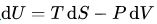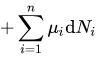where dU is the infinitesimal change of internal energy U, dS the infinitesimal change of entropy S, and dV is the infinitesimal change of volume V for a thermodynamic system in thermal equilibrium, and dNi is the infinitesimal change of particle number Ni of species i as particles are added or subtracted. T is absolute temperature, S is entropy, P is pressure, and V is volume. Other work terms, such as those involving electric and magnetic fields may be added.

From the above equation the chemical potential is given by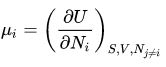This is an inconvenient expression for condensed matter systems, such as chemical solutions, as it is hard to control the volume and entropy to be constant while particles are added. A more convenient expression may be obtained by making a Legendre transformation to another thermodynamic potential: the Gibbs free energy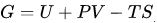. The differential of this is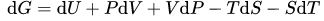and using the above expression for dU a differential relation for dG is obtained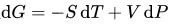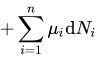As a consequence, another expression for μi results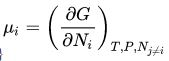and the change in Gibbs free energy of a system that is held at constant temperature and pressure is simply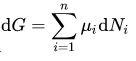In thermodynamic equilibrium, when the system concerned is at constant temperature and pressure but can exchange particles with its external environment, the Gibbs free energy is at its minimum for the system, that is  dG = 0 It follows that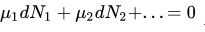Use of this equality provides the means to establish the equilibrium constant for a chemical reaction.

By making further Legendre transformations from U to other thermodynamic potentials like the Enthalpy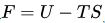and Helmholtz free energy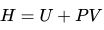, expressions for the chemical potential may be obtained in terms of these: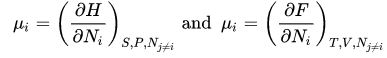.

These different forms for the chemical potential are all equivalent, meaning that they have the same physical content, and may be useful in different physical situations.

Applications

The Gibbs–Duhem equation is useful because it relates individual chemical potentials. For example, in a binary mixture, at constant temperature and pressure, the chemical potentials of the two participants are related byEvery instance of phase or chemical equilibrium is characterized by a constant. For instance, the melting of ice is characterized by a temperature, known as the melting point at which solid and liquid phases are in equilibrium with each other. Chemical potentials can be used to explain the slopes of lines on a phase diagram by using the Clapeyron equation, which in turn can be derived from the Gibbs–Duhem equation. They are used to explain colligative properties such as melting-point depression by the application of pressure. Both Raoult's law and Henry's law can be derived in a simple manner using chemical potentials.

History

Chemical potential was first described by the American engineer, chemist and mathematical physicist Josiah Willard Gibbs. He defined it as follows:

If to any homogeneous mass in a state of hydrostatic stress we suppose an infinitesimal quantity of any substance to be added, the mass remaining homogeneous and its entropy and volume remaining unchanged, the increase of the energy of the mass divided by the quantity of the substance added is the potential for that substance in the mass considered.

Gibbs later noted[citation needed] also that for the purposes of this definition, any chemical element or combination of elements in given proportions may be considered a substance, whether capable or not of existing by itself as a homogeneous body. This freedom to choose the boundary of the system allows chemical potential to be applied to a huge range of systems. The term can be used in thermodynamics and physics for any system undergoing change. Chemical potential is also referred to as partial molar Gibbs energy (see also partial molar property). Chemical potential is measured in units of energy/particle or, equivalently, energy/mole.

In his 1873 paper A Method of Geometrical Representation of the Thermodynamic Properties of Substances by Means of Surfaces, Gibbs introduced the preliminary outline of the principles of his new equation able to predict or estimate the tendencies of various natural processes to ensue when bodies or systems are brought into contact. By studying the interactions of homogeneous substances in contact, i.e. bodies, being in composition part solid, part liquid, and part vapor, and by using a three-dimensional volume–entropy–internal energy graph, Gibbs was able to determine three states of equilibrium, i.e. "necessarily stable", "neutral", and "unstable", and whether or not changes will ensue. In 1876, Gibbs built on this framework by introducing the concept of chemical potential so to take into account chemical reactions and states of bodies that are chemically different from each other. In his own words, to summarize his results in 1873, Gibbs states:

If we wish to express in a single equation the necessary and sufficient condition of thermodynamic equilibrium for a substance when surrounded by a medium of constant pressure P and temperature T, this equation may be written: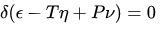where δ refers to the variation produced by any variations in the state of the parts of the body, and (when different parts of the body are in different states) in the proportion in which the body is divided between the different states. The condition of stable equilibrium is that the value of the expression in the parenthesis shall be a minimum.

In this description, as used by Gibbs, ε refers to the internal energy of the body, η refers to the entropy of the body, and ν is the volume of the body.

Offer running on EduRev: Apply code STAYHOME200 to get INR 200 off on our premium plan EduRev Infinity!

159 docs

,

,

,

,

,

,

,

,

,

,

,

,

,

,

,

,

,

,

,

,

,

,

,

,

;